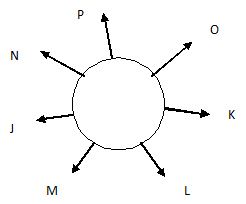# Schneider Reasoning Question

DIRECTIONS for the question 1 to 4:In this question, relationship between different elements is shown in the statement. The statement is followed by two conclusions. Study the conclusions based on the given statement and select the appropriate answer.
1. Statements: I = N ≥ C = L ≤ U = D
Conclusion I: I = D
Conclusion II: D < I
1. Neither conclusion I nor II is true
2. Only conclusion I is true
3. Both conclusion I and II are true
4. Either conclusion I or II is true
5. Only conclusion II is true
I= N ≥ C = L ≤ U = D
Conclusion I: I = D, is false
Conclusion II: D < I, is false.
Neither conclusion I nor conclusion II is true.
2. Statement: S<W = I<T = C<H
Conclusion I: C>S
Conclusion II: H > W
1. Neither conclusion I nor II is true
2. Only conclusion I is true
3. Both conclusion I and II are true
4. Either conclusion I or II is true
5. Only conclusion II is true
S < W = I < T = C < H
Conclusion I: C>S, is true.
Conclusion II:H > W, is true.
Both conclusion I & conclusion II is true.
1. Statement: D<I = A<R = E>S
Conclusion I: E > D
Conclusion II: I > S
1. Neither conclusion I nor II is true
2. Only conclusion I is true
3. Both conclusion I and II are true
4. Either conclusion I or II is true
5. Only conclusion II is true
D < I = A < R = E > S
Conclusion I: E > D, is true.
Conclusion II: I > S, is false
Only Conclusion I is true.
2. Statement: A>N<G = E<L>S
Conclusion I: A>S
Conclusion II: L > N
1. Neither conclusion I nor II is true
2. Only conclusion I is true
3. Both conclusion I and II are true
4. Either conclusion I or II is true
5. Only conclusion II is true
A > N < G = E < L > S
Conclusion I A > S, is false.
Conclusion II: L > N, is true.
Only Conclusion II is true.
DIRECTIONS for the questions 5 - 8:Study the following information and answer the following question.
Seven people- J, K, L, M, N, O and P are sitting around a circular table facing the centre with equal distance between them but not necessarily in the same order. O sits second to the right of L. Only three people sit between O and M. P sits second to the left of J. J is not an immediate neighbour of O. Only one person sits between P and K.
3. What is the position of P with respect to L?
1. Third to the right
2. Immediate right
3. Second to the left
4. Third to the left
5. Immediate leftP sits third to the right of L.
4. Which of the following statements is not true with respect to N as per the given arrangement?
1. Only one people sit between N and O.
2. P is an immediate neighbor of N
3. N sits to the immediate left of J.
4. M sits second to the right of N.
5. All the given statements are trueAll the given statements in options are true.
5. In which of the following groups of persons, is the third person sitting exactly between the first and second person as per the given arrangement?
1. LPK
2. PON
3. JML
4. KML
5. NPJIn KML, third person sitting exactly between the first and second person as per the given arrangement.
6. Who sits fourth to the right of K?
1. O
2. L
3. N
4. J
5. Other than those given as optionsJ sits fourth to the right of K.
7. If only one meaningful English word can be made with the fourth, the sixth and the ninth letters of the word  COMPUTERISE , {when counted from left to right) using each letter only once, then which of the following will be the second letter of the word from the right. If no such word can be formed, then your answer is 'X'. If more than one such word can be formed then your answer is 'Z'?
1. X
2. P
3. Z
4. I
5. None of these